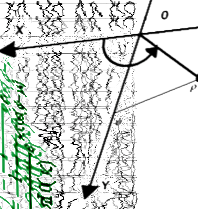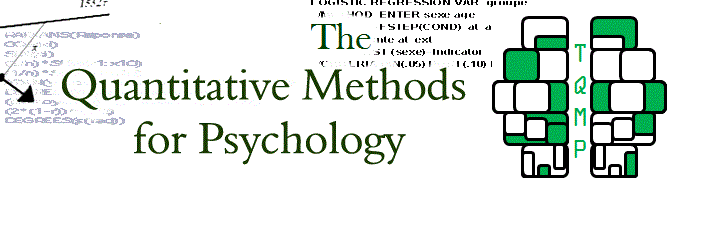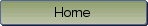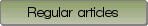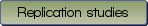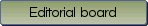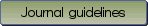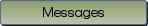The assumption of sphericity in repeated-measures designs: What it means and what to do when it is violated

Bibliographic information: BibTEX format RIS format XML format APA style
Cited references information: BibTEX format APA style

Lane, David M.
114-122
Keywords: analysis of variance , sphericity , epsilon correction , repeated measures
(no sample data)   (Appendix)

Violating the assumption of sphericity in repeated-measures analysis of variance leads to an inflated Type I error rate. The first portion of this article provides a thorough yet non-technical description of the sphericity assumption and explains why violations of sphericity lead to an inflated Type I error rate. The second portion describes univariate and multivariate approaches for addressing the problem of an inflated Type I error rate. The univariate approach involves estimating the parameter $\varepsilon$ that reflects the degree to which sphericity is violated and then reducimg the degrees of freedom by multiplying them by the estimate of $\varepsilon$. Two estimates of $\varepsilon$, $\mathaccentV{hat}05E\varepsilon$ and $\mathaccentV{tilde}07E\varepsilon$, have been recommended. The former has lower power than the latter whereas the latter fails to fully control the Type I error rate under some circumstances. The multivariate approach does not assume sphericity and therefore does not have an inflated Type I error rate. A decision tree for deciding among $\mathaccentV{hat}05E\varepsilon$, $\mathaccentV{tilde}07E\varepsilon$, and the multivariate approach based on a review of previously published simulations is presented along with a JavaScript program to automate the navigation of the decision tree.

Be informed of the upcoming issues with RSS feed:RSS